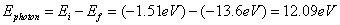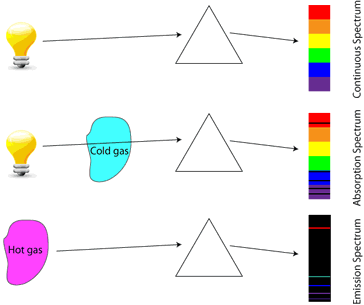# Models of the Atom

In the early 1900s, scientists around the world began to refine and revise our understanding of atomic structure and sub-atomic particles. Scientists understood that matter was made up of atoms, and J.J. Thompson had shown that atoms contained very small negative particles known as electrons, but beyond that, the atom remained a mystery.### Rutherford Model

New Zealand scientist Ernest Rutherford devised an experiment to better understand the rest of the atom. The experiment, known as Rutherford's Gold Foil Experiment, involved shooting alpha particles (helium nuclei) at a very thin sheet of gold foil, and observing the deflection of the particles after passing through the gold foil. Rutherford found that although most of the particles went through undeflected, a significant number of alpha particles were deflected by large amounts. Using an analysis based around Coulomb's Law and the conservation of momentum, Rutherford concluded that:1. Atoms have a small, massive, positive nucleus at the center.
2. Electrons must orbit the nucleus.
3. Most of the atom is made up of empty space.

Rutherford's model was incomplete, though, in that it didn't account for a number of effects predicted by classical physics. Classical physics predicted that if the electron orbits the atom, it is constantly accelerating, and should therefore emit photons of EM radiation. Because the atom emits photons, it should be losing energy, therefore the orbit of the electron would quickly decay into the nucleus and the atom would be unstable. Further, elements were found to emit and absorb EM radiation only at specific frequencies, which did not correlate to Rutherford's theory.### Bohr Model

Following Rutherford's discovery, Danish physicist Niels Bohr traveled to England to join Rutherford's research group and refine Rutherford's model of the atom. Instead of focusing on all atoms, Bohr confined his research to developing a model of the simple hydrogen atom. Bohr's model made the following assumptions:

1. Electrons don't lose energy as they accelerate around the nucleus. Instead, energy is quantized... electrons can only exist at specific discrete energy levels.
2. Each atom allows only a limited number of specific orbits at each energy level.
3. To change energy levels, an electron must absorb or emit a photon of energy exactly equal to the difference between the electron's initial and final energy levels:Question: Calculate the energy of the emitted photon when an electron moves from an energy level of -1.51 eV to -13.6 eV.

Answer:Question: What is the emitted photon's wavelength?

Answer:Bohr's Model, therefore, was able to explain the first two limitations of Rutherford's Model. Further, Bohr was able to use his model to predict the frequencies of photons emitted and absorbed by hydrogen, explaining Rutherford's problem of emission and absorption spectra! For his work, Bohr was awarded the Nobel Prize in Physics in 1922.

"If quantum mechanics hasn't profoundly shocked you, you haven't understood it yet." -- Niels Bohr

### Energy Level Diagrams

A useful tool for visualizing the allowed energy levels in an atom is the energy level diagram. Two of these diagrams (one for hydrogen and one for mercury) are provided for you below. In each of these diagrams, the n=1 energy state is the lowest possible energy for an electron of that atom, known as the ground state. The energy corresponding to n=1 is shown on the right side of the diagram in electron-volts. So, for hydrogen, the ground state is a level of -13.6 eV.

The energy levels are negative to indicate that the electron is bound by the nucleus of the atom. If the electron reaches 0 eV, it is no longer bound by the atom and can be emitted as a photoelectron (i.e. the atom becomes ionized). Any remaining energy becomes the kinetic energy of the photoelectron.Question: An electron in a hydrogen atom drops from the n=3 to the n=2 state. Determine the energy of the emitted radiation.

Answer:Question: What is the wavelength of the emitted radiation?

Answer:### Atomic Spectra

Once you understand the energy level diagram, it quickly becomes obvious that atoms can only emit certain frequencies of photons, correlating to the difference between energy levels as an electron falls from a higher energy state to a lower energy state. In similar fashion, electrons can only absorb photons with energy equal to the difference in energy levels as the electron jumps from a lower to a higher energy state. This leads to unique atomic spectra of emitted radiation for each element.An object that is heated to the point where it glows (incandescence) emits a continuous energy spectrum, described as blackbody radiation.

If a gas-discharge lamp is made from mercury vapor, the mercury vapor is made to emit light by application of a high electrical potential. The light emitted by the mercury vapor is created by electrons in higher energy states falling to lower energy states, therefore the photons emitted correspond directly in wavelength to the difference in energy levels of the electrons. This creates a unique spectrum of frequencies which can be observed by separating the colors using a prism, known as an emission spectrum. By analyzing the emission spectra of various objects, scientists can determine the composition of those objects.

In similar fashion, if light of all colors is shone through a cold gas, the gas will only absorb the frequencies corresponding to photon energies exactly equal to the difference between the gas's atomic energy levels. This creates a spectrum with all colors except those absorbed by the gas, known as an absorption spectrum.